Next: Heisenberg Equation of Motion Up: Quantum Dynamics Previous: Quantum Dynamics

# Schrödinger Equation of Motion

Up to now, we have only considered systems at one particular instant of time. Let us now investigate the time evolution if quantum mechanical systems.

Consider a system in a state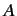that evolves in time. At time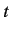, the state of the system is represented by the ket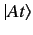. The labelis needed to distinguish this ket from any other ket (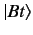, say) that is evolving in time. The labelis needed to distinguish the different states of the system at different times.

The final state of the system at timeis completely determined by its initial state at time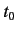plus the time interval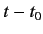(assuming that the system is left undisturbed during this time interval). However, the final state only determines the direction of the final state ket. Even if we adopt the convention that all state kets have unit norms, the final ket is still not completely determined, because it can be multiplied by an arbitrary phase-factor. However, we expect that if a superposition relation holds for certain states at timethen the same relation should hold between the corresponding time-evolved states at time, assuming that the system is left undisturbed between timesand. In other words, if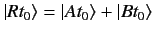(220)

for any three kets then we should have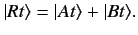(221)

This rule determines the time-evolved kets to within a single arbitrary phase-factor to be multiplied into all of them. The evolved kets cannot be multiplied by individual phase-factors because this would invalidate the superposition relation at later times.

According to Equations (220) and (221), the final ket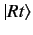depends linearly on the initial ket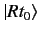. Thus, the final ket can be regarded as the result of some linear operator acting on the initial ket: i.e.,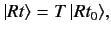(222)

whereis a linear operator that depends only on the timesand. The arbitrary phase-factor by which all time-evolved kets may be multiplied results in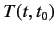being undetermined to an arbitrary multiplicative constant of modulus unity.

Because we have adopted a convention in which the norm of any state ket is unity, it make sense to define the time evolution operatorin such a manner that it preserves the length of any ket upon which it acts (i.e., if a ket is properly normalized at timethen it will remain normalized at all subsequent times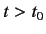). This is always possible, because the length of a ket possesses no physical significance. Thus, we require that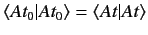(223)

for any ket, which immediately yields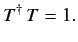(224)

Hence, the time evolution operatoris unitary.

Up to now, the time evolution operatorlooks very much like the spatial displacement operator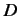introduced in the previous section. However, there are some important differences between time evolution and spatial displacement. In general, we do expect the expectation value of some observable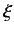to evolve with time, even if the system is left in a state of undisturbed motion (after all, time evolution has no meaning unless something observable changes with time). The triple product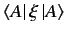can evolve either because the ket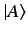evolves and the operatorstays constant, the ketstays constant and the operatorevolves, or both the ketand the operatorevolve. Because we are already committed to evolving state kets, according to Equation (222), let us assume that the time evolution operatorcan be chosen in such a manner that the operators representing the dynamical variables of the system do not evolve in time (unless they contain some specific time dependence).

We expect, from physical continuity, that if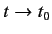then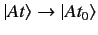for any ket. Thus, the limit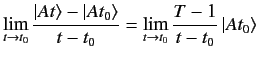(225)

should exist. Note that this limit is simply the derivative of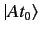with respect to. Let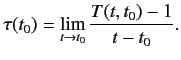(226)

It is easily demonstrated from Equation (224) that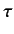is anti-Hermitian: i.e.,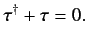(227)

The fact thatcan be replaced by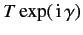(whereis real) implies thatis undetermined to an arbitrary imaginary additive constant (see Section 2.8). Let us define the Hermitian operator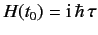. This operator is undetermined to an arbitrary real additive constant. It follows from Equations (225) and (226) that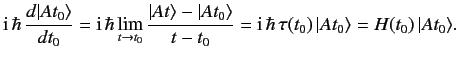(228)

When written for general, this equation becomes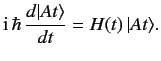(229)

Equation (229) gives the general law for the time evolution of a state ket in a scheme in which the operators representing the dynamical variables remain fixed. This equation is denoted the Schrödinger equation of motion. It involves a Hermitian operator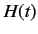which is, presumably, a characteristic of the dynamical system under investigation.

We saw, in Section 2.8, that if the operator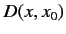displaces the system along the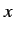-axis from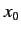tothen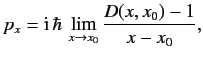(230)

where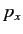is the operator representing the momentum conjugate to. Furthermore, we have just shown that if the operatorevolves the system in time fromtothen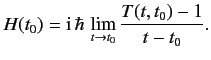(231)

Thus, the dynamical variable corresponding to the operator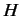stands to timeas the momentumstands to the coordinate. By analogy with classical physics, this suggests thatis the operator representing the total energy of the system. (Recall that, in classical physics, if the equations of motion of a system are invariant under an-displacement of the system then this implies that the system conserves momentum in the-direction. Likewise, if the equations of motion are invariant under a temporal displacement then this implies that the system conserves energy.) The operatoris usually called the Hamiltonian of the system. The fact that the Hamiltonian is undetermined to an arbitrary real additive constant is related to the well-known phenomenon that energy is undetermined to an arbitrary additive constant in physics (i.e., the zero of potential energy is not well-defined).

Substituting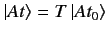into Equation (229) yields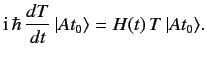(232)

Because this must hold for any initial state, we conclude that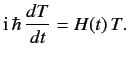(233)

This equation can be integrated to give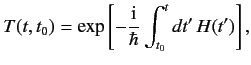(234)

where use has been made of Equations (224) and (225). (Here, we assume that Hamiltonian operators evaluated at different times commute with one another.) The fact thatis undetermined to an arbitrary real additive constant leavesundetermined to a phase-factor. Incidentally, in the above analysis, time is not an operator (we cannot observe time, as such), it is just a parameter (or, more accurately, a continuous label).Next: Heisenberg Equation of Motion Up: Quantum Dynamics Previous: Quantum Dynamics
Richard Fitzpatrick 2013-04-08